# Samacheer Kalvi Books: Tamilnadu State Board Text Books Solutions

## Samacheer Kalvi 10th Maths Chapter 7 Mensuration Notes PDF Download: Tamil Nadu STD 10th Maths Chapter 7 Mensuration NotesSamacheer Kalvi 10th Maths Chapter 7 Mensuration Notes PDF Download: Tamil Nadu STD 10th Maths Chapter 7 Mensuration Notes

We bring to you specially curated Samacheer Kalvi 10th Maths Chapter 7 Mensuration Notes PDF which have been prepared by our subject experts after carefully following the trend of the exam in the last few years. The notes will not only serve for revision purposes, but also will have several cuts and easy methods to go about a difficult problem.

 Board Tamilnadu Board Study Material Notes Class Samacheer Kalvi 10th Maths Subject 10th Maths Chapter Chapter 7 Mensuration Format PDF Provider Samacheer Kalvi Books

## How to Download Samacheer Kalvi 10th Maths Chapter 7 Mensuration Notes PDFs?

2. Click on the Samacheer Kalvi 10th Maths Notes PDF.
3. Look for your preferred subject.
4. Now download the Samacheer Kalvi 10th Maths Chapter 7 Mensuration notes PDF.

## Tamilnadu Samacheer Kalvi 10th Maths Solutions Chapter 7 Mensuration Unit Exercise 7

Question 1.
The barrel of a fountain-pen cylindrical in shape, is 7 cm long and 5 mm in diameter. A full barrel of ink in the pen will be used for writing 330 words on an average. How many words can be written using a bottle of ink containing one fifth of a litre?
Solution: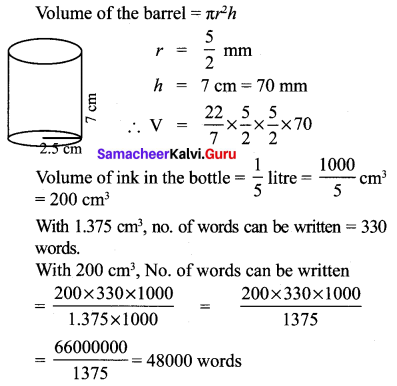Question 2.
A hemi-spherical tank of radius 1.75 m is full of water. It is connected with a pipe which empties the tank at the rate of 7 litre per second. How much time will it take to empty the tank completely?
Solution:
Suppose the pipe takes x seconds to empty the tank. Then, volume of the water that flows out of the tank in x seconds = Volume of the hemispherical tank.
Volume of the water that flows out of the tank in x seconds.
= Volume of hemispherical shell of radius 175 cm.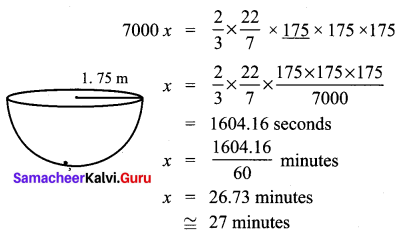Question 3.
Find the maximum volume of a cone that can be carved out of a solid hemisphere of radius r units.
Solution:
Radius of the base of cone = Radius of the hemisphere = r
Height of the cone = Radius of the hemisphere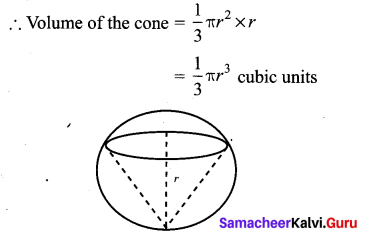Question 4.
An oil funnel of tin sheet consists of a cylindrical portion 10 cm long attached to a frustum of a cone. If the total height is 22 cm, the diameter of the cylindrical portion be 8cm and the diameter of the top of the funnel be 18 cm, then find the area of the tin sheet required to make the funnel.
Solution:
Slant height of the frustum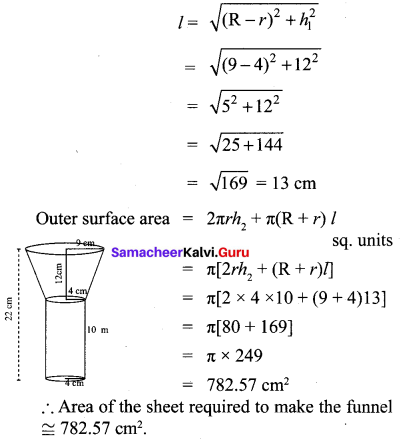Question 5.
Find the number of coins, 1.5 cm in diameter and 2 mm thick, to be melted to form a right circular cylinder of height 10 cm and diameter 4.5 cm.
Solution:
No. of coins required .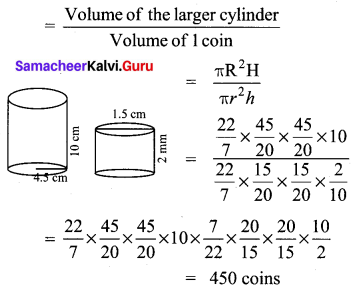Question 6.
A hollow metallic cylinder whose external radius is 4.3 cm and internal radius is 1.1 cm
and whole length is 4 cm is melted and recast into a solid cylinder of 12 cm long. Find the diameter of solid cylinder.
Solution:
Volume of the solid cylinder = Volume of the hollow cylinder melted.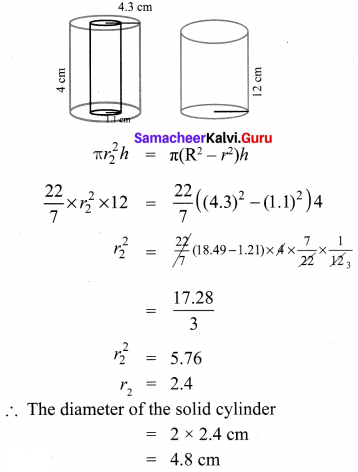Question 7.
The slant height of a frustum of a cone is 4 m and the perimeter of circular ends are 18 m and 16 m. Find the cost of painting its curved surface area at ₹ 100 per sq. m.
Solution: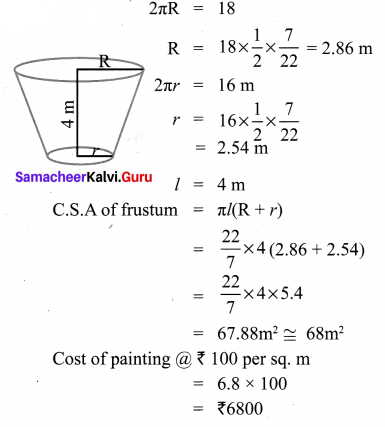Question 8.
A hemi-spherical hollow bowl has material of volume 436π3 cubic cm. Its external diameter is 14 cm. Find its thickness.
Solution: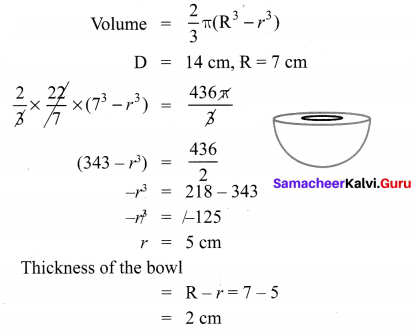Question 9.
The volume of a cone is 100557 cu. cm. The area of its base is 20117 sq. cm. Find the slant height of the cone.
Solution: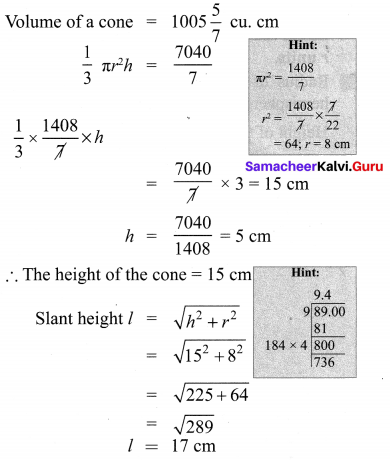Question 10.
A metallic sheet in the form of a sector of T a circle of radius 21 cm has central angle of 216°. The sector is made into a cone by bringing the bounding radii together. Find the volume of the cone formed.
Solution: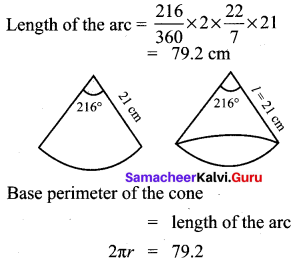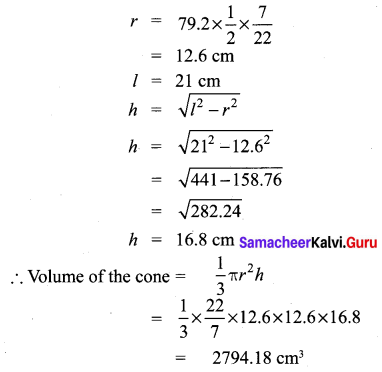## Tamilnadu Samacheer Kalvi 10th Maths Solutions Chapter 7 Mensuration Additional Questions

Question 1.
If the radii of the circular ends of a conical bucket which is 45 cm high are 28 cm and 7 cm, find the capacity of the bucket. (Use π=227)
Solution:
Clearly bucket forms frustum of a cone such that the radii of its circular ends are r1 = 28 cm, r2 = 7 cm, h = 45 cm.
Capacity of the bucket = volume of the frustum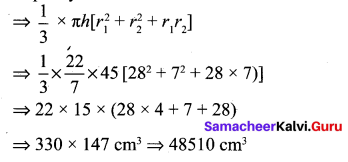Question 2.
Find the depth of a cylindrical tank of radius 28 m, if its capacity is equal to that of a rectangular tank of size 28 m × 16 m × 11 m.
Solution:
Volume of the cylindrical tank = Volume of the rectangle tank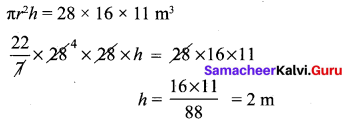Question 3.
What is the ratio of the volume of a cylinder, a cone, and a sphere. If each has the same diameter and same height?
Solution: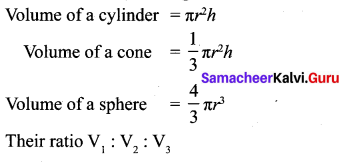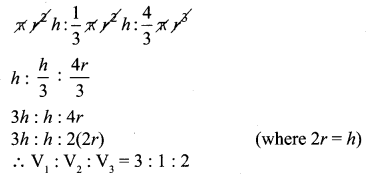Question 4.
Find the number of coins, 1.5 cm is diameter and 0.2 cm thick, to be melted to form a right circular cylinder of height 10 cm and diameter 4.5 cm.
Solution: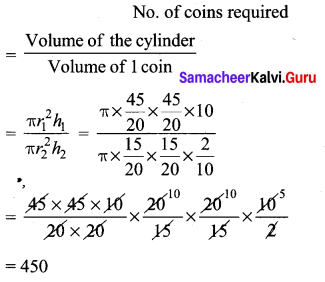Question 5.
A spherical ball of iron has been melted and made into small balls. If the raidus of each smaller ball is one-fourth of the radius of the original one, how many such balls can be made?
Solution: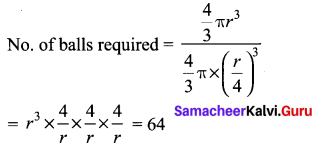Question 6.
A wooden article was made by scooping out a hemisphere from each end of a cylinder as shown in figure. If the height of the cylinder is 10cm and its base is of radius 3.5 cm find the total surface area of the article.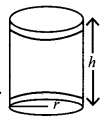Solution:
Radius of the cylinder be r Height of the cylinder be h Total surface area of the article = CSA of cylinder + CSA of 2 hemispheres = 2πrh + 2πr2 = 2πr (h + 2r)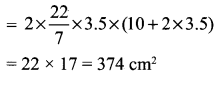## How to Prepare using Samacheer Kalvi 10th Maths Chapter 7 Mensuration Notes PDF?

Students must prepare for the upcoming exams from Samacheer Kalvi 10th Maths Chapter 7 Mensuration Notes PDF by following certain essential steps which are provided below.

• Use Samacheer Kalvi 10th Maths Chapter 7 Mensuration notes by paying attention to facts and ideas.
• Pay attention to the important topics
• Refer TN Board books as well as the books recommended.
• Correctly follow the notes to reduce the number of questions being answered in the exam incorrectly
• Highlight and explain the concepts in details.

## Frequently Asked Questions on Samacheer Kalvi 10th Maths Chapter 7 Mensuration Notes

#### How to use Samacheer Kalvi 10th Maths Chapter 7 Mensuration Notes for preparation??

Read TN Board thoroughly, make separate notes for points you forget, formulae, reactions, diagrams. Highlight important points in the book itself and make use of the space provided in the margin to jot down other important points on the same topic from different sources.

#### How to make notes for Samacheer Kalvi 10th Maths Chapter 7 Mensuration exam?

Read from hand-made notes prepared after understanding concepts, refrain from replicating from the textbook. Use highlighters for important points. Revise from these notes regularly and formulate your own tricks, shortcuts and mnemonics, mappings etc.
Share: